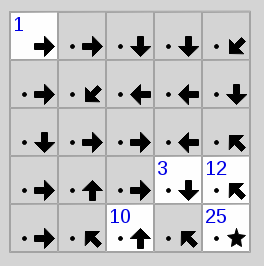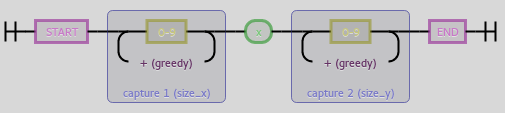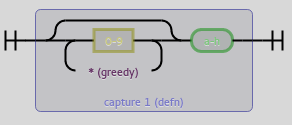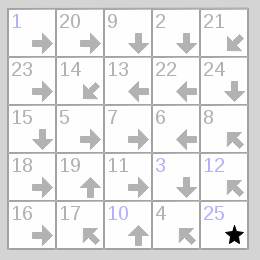# Other articles

1. Published: Thu 23 January 2020

# Podcast Time Machine

Recently one of my favourite podcasts ended after seven years. I have only been listening to this podcast for two years though. There is five year backlog for me to listen to. But there are many other podcasts I want to listen to and I do like the anticipation of waiting for a new episode.

So I built the Podcast Time Machine (github) where you can enter a podcast url and a delay and you will get a new link where every podcast episode is delayed by that many days and all episodes that would lie in the future are filtered out. So stuff happpening a week in podcast time are happpening in a week of real time.

I am looking forward to my first episode of harmontown, when it comes out after a seven-year delay. Feel free to subscribe to your own delayed podcasts. I do not keep access logs so listen to your favorite stuff again (and again).

2. Published: Mon 21 March 2016

# Solving Tatham's Puzzles - Signpost (backtracking)

I've started writing solvers for games of Simon Tatham's Portable Puzzle Collection. Those Problems can usually be reduced to some NP-complete problem. This is quite a fun exercise.Unsolved Signpost Puzzle

We will be continuing with the puzzle "Signpost", which is a variant of Hamiltonian paths in a directed graph where, for some fields or nodes, the position within the path is given.

The goal of the puzzle is to connect the Node "1" to some node, which is then node "2" in the direction the arrow points to. All numbers need to be connected to their successor until all nodes have a single unique number from "1" to (in this example) "25".

We will be solving this problem in two different ways, both with the help of the programming language Python. But first of all we'll need to convert a puzzle into a machine readable format. Luckily, Signpost has an easy and accessible format. The problem instance from the image is the instance 5x5:1cceefcfggeeccghcac3e12hch10ah25a. It starts off with the size of the problem and then a list of letters that show the directions of the arrow where a is north or 12 o'clock and all other letters go clockwise with h being north-west. A letter can be prefixed by a number which is the hint for that node. That node's number is fixed. The cells or nodes are defined line by line from the top left to the bottom right.

Problems don't need to start with 1 or end with the last number. The start and the end of the path can be anywhere but puzzles generated with the ends at opposite corner look nicer.

First we define what our data looks like. We implement a simple form of directed graph that we define as a set of nodes and their successors. From that we derive following definition:

```class Node(object):
hint = None
following = frozenset()

def __init__(self, direction):
self.direction = direction
```

We don't want to use any of that code in a library or something something so we don't do anything fancy here and we use the simplest class definition that comes to mind.^(?P<size_x>\d+)x(?P<size_y>\d+)\$(?P<defn>\d*[a-h])

Now we go on to parsing the problem. The parsing function should get the problem as a string and return a 3-tuple containing width and height of the problem as well as a mapping of positions within the grid to ``Node`` instances.

Onto actually parsing. We can split at the colon and then regular expressions to the rescue. The size definition has the form ^(?P<size_x>\d+)x(?P<size_y>\d+)\$ while a single node definition has the form (?P<defn>\d*[a-h]). As we can see, the digits are optional but we need to greedily accept them. All non-overlapping occurences in order will be our cell definitions.

In code we, again, do nothing too too fancy:

```def parse(puzzle_str):
directions = {
'a': (0,-1), 'b': (1,-1), 'c': (1,0), 'd': (1,1),
'e': (0,1),  'f': (-1,1), 'g': (-1,0),'h': (-1,-1)
}

size, _, definition = puzzle_str.partition(':')
r_size = re.compile(r"^(?P<size_x>\d+)x(?P<size_y>\d+)\$")
r_defn = re.compile(r"(?P<defn>\d*[a-h])")
size_t = tuple(map(int, r_size.match(size).groups()))
w, h = size_t
nodes = {}
for n, m in enumerate(r_defn.finditer(definition)):
pos = (n % w, n // w)
nodes[pos] = Node(directions[m.group(0)[-1:]])
hint = m.group(0)[:-1]
if hint:
nodes[pos].hint = int(hint)
return w, h, nodes
```

And we also need to fill in the successor Nodes. This is no problem at all.

```w, h, nodes = parse(sys.argv)
from itertools import product
for x, y in product(range(w), range(h)):
this_node = nodes[(x,y)]
dx, dy = this_node.direction
x, y = x + dx, y + dy
while x >= 0 and x < w and y >= 0 and y < h:
this_node.following = this_node.following | frozenset([nodes[x,y]])
x, y = x + dx, y + dy
```

And now on to actually solving this thing. The idea is, that we slowly build a list of Nodes that is our solution. The first element is the Node with the hint "1" and we iterate through all nodes that follow that Node and do not have a hint that is other than "2", and so on. And of course, the Node that we add to the solution is not allowed to have already been part of the solution and if there is a node with that hint, it should be this exact one. If we find such a conflict in our solution, we reject that solution and return to an earlier partial solution.

Instead of using recursion, we build a queue of partial solutions until we get a complete solution. This is functionally identical to restarting a solver function with partial solutions of increasing size. In that case, the call stack manages the backtracking. But we'll do it with a queue this time. We build the queue so that every element in the list is either

1. a partial solution (beginning from 1) without conflicts
2. a partial solution (beginning from 1) where the last element is in some conflict
3. a complete solution

For case 1, we remove the partial solution that has the length n and add all partial solutions of length n+1 by iterating through all successor Nodes that have not yet been part of the partial solution. For case 2, we reject that partial solution. For case 3, we immediately return that solution and are finished.

```def solve_dhc(nodes):
# get the Node that has hint 1
first = next(iter(n for n in nodes.values() if n.hint == 1))
# get the set of all hints, so it is easier to check for conflicts
hints = frozenset(n.hint for n in nodes.values() if n.hint is not None)
from collections import deque
q = deque([[first]]) # initializing queue
while q:
curr = q.pop()
idx = len(curr)
if (idx in hints or curr[-1].hint is not None) and curr[-1].hint != idx:
continue # case 2
if idx == len(nodes):
return curr # case 3
for _next in curr[-1].following: # case 1
if _next not in curr:
q.append(curr + [_next])
```

This algorithm terminates because we always remove one element from the queue of some length n and possibly add a large but finite amount of elements of length n+1 and the algorithm terminates when we have an element in the queue of some possibly large but finite length.

Sidenote: if you use popleft instead of pop you get a breadth-first search instead the depth-first search, that is implemented here. Add to the right and pop from the right for depth-first and add to the right and pop from the left for breadth-first. Since every proper solution has the exact same length/depth (that we know and never go further anyways), searching depth-first is strictly better than breadth-first.

And given a solution, we still have to print it. That's the easy part.

```dhc = solve_dhc(nodes)

fill = len(str(w*h))
for l in range(h):
for c in range(w):
node = nodes[(c,l)]
print(str(dhc.index(node) + 1).rjust(fill), end=" ")
print("")
```Solved Puzzle

And there's the solution for our puzzle.

```\$ python3 signpost_dhc.py 5x5:1cceefcfggeeccghcac3e12hch10ah25a
1 20  9  2 21
23 14 13 22 24
15  5  7  6  8
18 19 11  3 12
16 17 10  4 25
```

Find the code here: github.com/maweki/tatham-slv

3. Published: Thu 12 November 2015

# talk at Leipzig's Haskell conference HaL-10

As I said before: I will hold a small talk about dogelang at Leipzig's next Haskell conference HaL-10.

Here's a translation of the abstract (original in German):

dg - The better Haskell is named Python

Who needs typeclasses? Or static type system even? Why have side-effects to be so complicated? And what are monads?

There must be something better!

dg is Python with Haskell syntax. In a short talk (15 minutes) I want to introduce, on a funny note, the "better Haskell".

Under the slogan "what we don't have, you won't need" we will be looking at features of the Haskell language and their counterparts in dg. To conclude, we will take a look at how intelligent a Python program can look, if it has the proper syntax.

4. Published: Thu 12 November 2015

# Deciding on the pure-ness of a python function

Through the work with dogelang I am currently looking at pureness of python functions and on how to decide and annotate pureness so that I, in theory, could write a static checker that checks whether the annotated functions are pure.

We can take the definition of pureness from the wikipedia and it is as follows

1. The function always evaluates the same result value given the same argument value(s). The function result value cannot depend on any hidden information or state that may change while program execution proceeds or between different executions of the program, nor can it depend on any external input from I/O devices.
2. Evaluation of the result does not cause any semantically observable side effect or output, such as mutation of mutable objects or output to I/O devices.

Python makes few restrictions on side-effects and mutability and since we even can override basic operators (like the dot-operator for attribute access), it is hard to infer anything remotely useful or non-trivial from a python-program.

I have been, for example, responsible for code like that (paracoded for your convenience):

```def cls(object):
def __init__(self):
self.d = {}

def __getattr__(self, attr)
if not attr in self.d:
self.d[attr] = someobj(attr)
return self.d[attr]

A = cls()
A.foo # this line has side effects
```

This violates the basic principle of python that an expression should either have a return value or a side-effect but not both. But we might have done this for good reason. In this case, the academic value of the "front-end" of the library outweighs the atrocities of the implementation. But it serves as a fine example that, it is hard to reason about even simple looking expressions, if they include the dot-operator (or any other operator for that matter), especially if you don't have the full class definition at hand.

But it isn't only user-made classes that don't hold true to that principle. Most low-level functions that interact with the operating system do that in some way. They have their side-effect within the realms of the rest of the system and still they have a return value that represents some aspect of the operation within our program.

os.write(fd, str)
Write the bytestring in str to file descriptor fd. Return the number of bytes actually written.
open(file, ...)
Open file and return a corresponding file object. If the file cannot be opened, an OSError is raised.

So we would want to mark any function as impure that has a reference to one of these impure functions. But we would also want to mark any functions defined within these impure functions to be themselves impure. Looking at the following code it would be hard to infere whether a function has a reference to, let's say 'open'.

```o, f = open, "file.tmp"
def fun():
o(f)
```

Given Rice's theorem, I'd think that properly tracking references would either be undecidable or at least a problem of exponential growth (tracking all possible assignments to some reference). So to be on the safe side, we state the following as our impurity rule number 1: A function is impure if it uses a reference from an impure function or has a reference to an impure function. We would also want an exhaustive list of builtin impure functions, applying the rules recursively.

In the former code example the surrounding block leaks (that's what we will call it) the reference to both o and f into the namespace of fun. This would not be a problem. We even want this behaviour. Let's look at this example:

```def f():
return x*x
x = 5
print(f()) # 25
x = 7
print(f()) # 49
```

This is contrary to our definition of purity, that the function, given the same arguments should evaluate to the same value. So this only happened, because the reference was updated. Let's add impurity rule number 2: A function is impure if a name is assigned twice and a reference to that name is leaked. This should cover all cases since shenanigans like the following cause a NameError.

```def a():
yield (lambda : x*x)
x = 3
yield None
x, i = 5, a()
g = next(i)
print(g()) # Name Error: free variable 'x' referenced before assignment in enclosing scope
next(i)
print(g())
```

Here we see the yield-keyword we have the case of suspended computation. The next-function is of course part of the set of impure builtin functions because it returns a value and advances the internal state of the generator. Since for rule 2 we introduced the leaked name, we can still allow multiple assignments within a function if the name is not leaked because we want to allow, for example, the generator-function infinity to be pure.

```def infinity():
i = 0
while True:
yield i
i = i + 1
```

Here, I find, we arrive at an impasse. Every iteration over a generator "uses the generator up". We could say that we won't allow references to generator-objects but that seems arbitrarily strict. Also, we can't really know which name could be used by a generator-object which would lead to not allowing any bare names (only function calls). Disallowing generators seems impossible since map and filter and other functional primitives depend on it.

I would welcome any ideas for a rule that would solve this issue of leaking the internal state of a generator.

Page 1 / 2 »